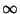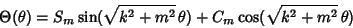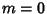## Helmholtz Differential Equation--Spherical Surface

On the surface of a Sphere, attempt Separation of Variables in Spherical Coordinates by writing(1)

then the Helmholtz Differential Equation becomes(2)

Dividing both sides by,(3)

which can now be separated by writing(4)

The solution to this equation must be periodic, somust be an Integer. The solution may then be defined either as a Complex function(5)

for, ...,, or as a sum of Real sine and cosine functions(6)

for, ...,. Plugging (4) into (3) gives(7)(8)

which is the Legendre Differential Equation forwith(9)

giving(10)(11)

Solutions are therefore Legendre Polynomials with a Complex index. The general Complex solution is then(12)

and the general Real solution is(13)

Note that these solutions depend on only a single variable. However, on the surface of a sphere, it is usual to express solutions in terms of the Spherical Harmonics derived for the 3-D spherical case, which depend on the two variablesand.